Chapter 13. Chemical Equilibrium

# Some Special Types of Equilibria

Learning Objectives

1. Identify several special chemical equilibria and construct their Ka expressions.

In one sense, all chemical equilibria are treated the same. However, there are several classes of reactions that are noteworthy because of either the identities of the reactants and products or the form of the Keq expression.

# Weak Acids and Bases

In Chapter 12 “Acids and Bases”, we noted how some acids and bases are strong and some are weak. If an acid or base is strong, it is ionized 100% in H2O. HCl(aq) is an example of a strong acid:

HCl(aq) → H+(aq)  +  Cl(aq) (100%)

However, if an acid or base is weak, it may dissolve in H2O but does not ionize completely. This means that there is an equilibrium between the unionized acid or base and the ionized form. HC2H3O2 is an example of a weak acid:

HC2H3O2 (aq) ⇄ H+(aq) + C2H3O2(aq)

HC2H3O2 is soluble in H2O (in fact, it is the acid in vinegar), so the reactant concentration will appear in the equilibrium constant expression. But not all the molecules separate into ions. This is the case for all weak acids and bases.

An acid dissociation constant, Ka, is the equilibrium constant for the dissociation of a weak acid into ions. Note the a subscript on the K; it implies that the substance is acting as an acid. The larger Ka is, the stronger the acid is. Table 13.1 “Acid Dissociation Constants for Some Weak Acids” lists several acid dissociation constants. Keep in mind that they are just equilibrium constants.

Table 13.1 Acid Dissociation Constants for Some Weak Acids
Acid Ka
HC2H3O2 1.8 × 10−5
HClO2 1.1 × 10−2
H2PO4 6.2 × 10−8
HCN 6.2 × 10−10
HF 6.3 × 10−4
HNO2 5.6 × 10−4
H3PO4 7.5 × 10−3

Note also that the acid dissociation constant refers to one H+ ion coming off the initial reactant. Thus the acid dissociation constant for H3PO4 refers to this equilibrium:

H3PO4(aq) ⇄ H+(aq) + H2PO4(aq)    K= 7.5×10−3

The H2PO4 ion, called the dihydrogen phosphate ion, is also a weak acid with its own acid dissociation constant:

H2PO4(aq) ⇄ H+(aq) + HPO42−(aq)    K= 6.2×10−8

Thus for so-called polyprotic acids, each H+ ion comes off in sequence, and each H+ ion that ionizes does so with its own characteristic Ka.

Example 13.10

Write the equilibrium equation and the Ka expression for HSO4 acting as a weak acid.

Solution
HSO4 acts as a weak acid by separating into an H+ ion and an SO42− ion:

HSO4(aq) ⇄ H+(aq) + SO42−(aq)

The Ka is written just like any other equilibrium constant, in terms of the concentrations of products divided by concentrations of reactants:

Ka = [H+][SO42−][HSO4]

Test Yourself
Write the equilibrium equation and the Ka expression for HPO42− acting as a weak acid.

HPO42−(aq) ⇄ H+(aq) + PO43−(aq)          Ka = [H+][PO43−][HPO42−]

The Ka is used in equilibrium constant problems just like other equilibrium constants are. However, in some cases, we can simplify the mathematics if the numerical value of the Ka is small, much smaller than the concentration of the acid itself. Example 13.11 illustrates this.

Example 13.11

What is the pH of a 1.00 M solution of HC2H3O2? The Ka of HC2H3O2 is 1.8 × 10−5.

Solution
This is a two-part problem. We need to determine [H+] and then use the definition of pH to determine the pH of the solution. For the first part, we can use an ICE chart:

HC2H3O2(aq) H+(g) + C2H3O2(g)
I 1.00 0 0
C x +x +x
E 1.00 − x +x +x

We now construct the Ka expression, substituting the concentrations from the equilibrium row in the ICE chart: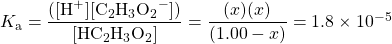Here is where a useful approximation comes in: at 1.8 × 10−5, HC2H3O2 will not ionize very much, so we expect that the value of x will be small. It should be so small that in the denominator of the fraction, the term (1.00 − x) will likely be very close to 1.00. As such, we would introduce very little error if we simply neglect the x in that term, making it equal to 1.00: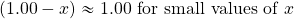This simplifies the mathematical expression we need to solve: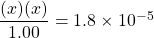This is much easier to solve than a more complete quadratic equation. The new equation to solve becomes: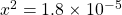Taking the square root of both sides: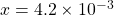Because x is the equilibrium concentrations of H+ and C2H3O2, we thus have: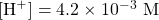Notice that we are justified by neglecting the x in the denominator; it truly is small compared to 1.00. Now we can determine the pH of the solution: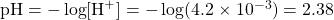Test Yourself
What is the pH of a 0.500 M solution of HCN? The Ka of HCN is 6.2 × 10−10.

4.75

Weak bases also have dissociation constants, labelled Kb (the b subscript stands for base). However, values of Kb are rarely tabulated because there is a simple relationship between the Kb of a base and the Ka of its conjugate acid: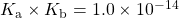Thus it is simple to calculate the Kb of a base from the Ka of its conjugate acid.

Example 13.12

What is the value of Kb for C2H3O2, which can accept a proton and act as a base?

Solution
To determine the Kb for C2H3O2, we need to know the Ka of its conjugate acid. The conjugate acid of C2H3O2 is HC2H3O2. The Ka for HC2H3O2 is in Table 13.1 “Acid Dissociation Constants for Some Weak Acids” and is 1.8 × 10−5. Using the mathematical relationship between Ka and Kb: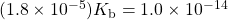Solving,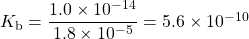Test Yourself
What is the value of Kb for PO43−, which can accept a proton and act as a base? The Ka for HPO42− is 2.2 × 10−13.

4.5 × 10−2

# Autoionization of Water

In Chapter 12 “Acids and Bases”, we introduced the autoionization of water — the idea that water can act as a proton donor and proton acceptor simultaneously. Because water is not a strong acid (Table 12.1 “Strong Acids and Bases”), it must be a weak acid, which means that its behaviour as an acid must be described as an equilibrium. That equilibrium is as follows:

H2O(ℓ) + H2O(ℓ) ⇄ H3O+(aq) + OH(aq)

The equilibrium constant includes [H3O+] and [OH] but not [H2O(ℓ)] because it is a pure liquid. Hence the expression does not have any terms in its denominator:

K = [H3O+][OH] ≡ Kw = 1.0 × 10−14

This is the same Kw that was introduced in Chapter 12 and the same 1.0 × 10−14 that appears in the relationship between the Ka and the Kb of a conjugate acid-base pair. In fact, we can rewrite this relationship as follows: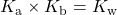# Insoluble Compounds

In Chapter 4 “Chemical Reactions and Equations”, in section “Types of Chemical Reactions: Single- and Double-Displacement Reactions”, on chemical reactions, the concept of soluble and insoluble compounds was introduced. Solubility rules were presented that allow a person to predict whether certain simple ionic compounds will or will not dissolve.

Describing a substance as soluble or insoluble is a bit misleading because virtually all substances are soluble; they are just soluble to different extents. In particular for ionic compounds, what we typically describe as an insoluble compound can actually be ever so slightly soluble; an equilibrium is quickly established between the solid compound and the ions that do form in solution. Thus the hypothetical compound MX does in fact dissolve but only very slightly. That means we can write an equilibrium for it:

MX(s) ⇄ M+(aq) + X(aq)

The equilibrium constant for a compound normally considered insoluble is called a solubility product constant. The equilibrium constant for a compound normally considered insoluble. and is labelled Ksp (with the subscript sp, meaning “solubility product”). Because the reactant is a solid, its concentration does not appear in the Ksp expression, so like Kw, expressions for Ksp do not have denominators. For example, the chemical equation and the expression for the Ksp for AgCl, normally considered insoluble, are as follows:

AgCl(s) ⇄ Ag+(aq) + Cl(aq)    Ksp = [Ag+][Cl]

Table 13.2 “Solubility Product Constants for Slightly Soluble Ionic Compounds” lists some values of the Ksp for slightly soluble ionic compounds.

Table 13.2 Solubility Product Constants for Slightly Soluble Ionic Compounds
Compound Ksp
BaSO4 1.1 × 10−10
Ca(OH)2 5.0 × 10−6
Ca3(PO4)2 2.1 × 10−33
Mg(OH)2 5.6 × 10−12
HgI2 2.9 × 10−29
AgCl 1.8 × 10−10
AgI 8.5 × 10−17
Ag2SO4 1.5 × 10−5

Example 13.13

Write the Ksp expression for Ca3(PO4)2.

Solution
Recall that when an ionic compound dissolves, it separates into its individual ions. For Ca3(PO4)2, the ionization reaction is as follows:

Ca3(PO4)2(s) ⇄ 3Ca2+(aq) + 2PO43–(aq)

Hence the Ksp expression is

Ksp = [Ca2+]3[PO43−]2

Test Yourself
Write the Ksp expression Ag2SO4.

Ksp = [Ag+]2[SO42−]

Equilibrium problems involving the Ksp can also be done, and they are usually more straightforward than other equilibrium problems because there is no denominator in the Ksp expression. Care must be taken, however, in completing the ICE chart and evaluating exponential expressions.

Example 13.14

What are [Ag+] and [Cl] in a saturated solution of AgCl? The Ksp of AgCl is 1.8 × 10−10.

Solution
The chemical equation for the dissolving of AgCl is

AgCl(s) ⇄ Ag+(aq) + Cl(aq)

The Ksp expression is as follows:

Ksp = [Ag+][Cl]

So the ICE chart for the equilibrium is as follows:

AgCl(s) Ag+(aq) + Cl(aq)
I 0 0
C x +x +x
E +x +x

Notice that we have little in the column under AgCl except the stoichiometry of the change; we do not need to know its initial or equilibrium concentrations because its concentration does not appear in the Ksp expression. Substituting the equilibrium values into the expression: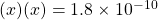Solving,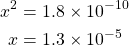Thus [Ag+] and [Cl] are both 1.3 × 10−5 M.

Test Yourself
What are [Ba2+] and [SO42−] in a saturated solution of BaSO4? The Ksp of BaSO4 is 1.1 × 10−10.

1.0 × 10−5 M

Example 13.15

What are [Ca2+] and [PO43−] in a saturated solution of Ca3(PO4)2? The Ksp of Ca3(PO4)2 is 2.1 × 10−33.

Solution
This is similar to Example 13.14, but the ICE chart is much different because of the number of ions formed.

Ca3(PO4)2(s) 3Ca2+(aq) + 2PO43−(aq)
I 0 0
C x +3x +2x
E +3x +2x

For every unit of Ca3(PO4)2 that dissolves, three Ca2+ ions and two PO43− ions are formed. The expression for the Ksp is also different:

Ksp = [Ca2+]3[PO43−]2 = 2.1 × 10−33

Now when we substitute the unknown concentrations into the expression, we get: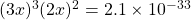When we raise each expression inside parentheses to the proper power, remember that the power affects everything inside the parentheses, including the number. So: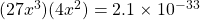Simplifying: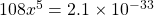Dividing both sides of the equation by 108, we get: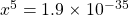Now we take the fifth root of both sides of the equation (be sure you know how to do this on your calculator):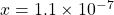We are not done yet. We still need to determine the concentrations of the ions. According to the ICE chart, [Ca2+] is 3x, not x. So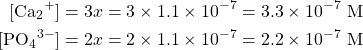Test Yourself
What are [Mg2+] and [OH] in a saturated solution of Mg(OH)2? The Ksp of Mg(OH)2 is 5.6 × 10−12.

[Mg2+] = 1.1 × 10−4 M; [OH] = 2.2 × 10−4 M

# Food and Drink App: Solids in Your Wine Bottle

People who drink wine from bottles (as opposed to boxes) will occasionally notice some insoluble materials in the wine, either crusting the bottle, stuck to the cork, or suspended in the liquid wine itself. The accompanying figure shows a cork encrusted with coloured crystals. What are these crystals?The red crystals on the top of the wine cork are from insoluble compounds that are not soluble in the wine.

One of the acids in wine is tartaric acid (H2C4H4O6). Like the other acids in wine (citric and malic acids, among others), tartaric acid imparts a slight tartness to the wine. Tartaric acid is rather soluble in H2O, dissolving over 130 g of the acid in only 100 g of H2O. However, the potassium salt of singly ionized tartaric acid, potassium hydrogen tartrate (KHC4H4O6; also known as potassium bitartrate and better known in the kitchen as cream of tartar), has a solubility of only 6 g per 100 g of H2O. Thus, over time, wine stored at cool temperatures will slowly precipitate potassium hydrogen tartrate. The crystals precipitate in the wine or grow on the insides of the wine bottle and, if the bottle is stored on its side, on the bottom of the cork. The colour of the crystals comes from pigments in the wine; pure potassium hydrogen tartrate is clear in its crystalline form, but in powder form it is white.

The crystals are harmless to ingest; indeed, cream of tartar is used as an ingredient in cooking. However, most wine drinkers don’t like to chew their wine, so if tartrate crystals are present in a wine, the wine is usually filtered or decanted to remove the crystals. Tartrate crystals are almost exclusively in red wines; white and rose wines do not have as much tartaric acid in them.

Key Takeaways

• Equilibrium constants exist for certain groups of equilibria, such as weak acids, weak bases, the autoionization of water, and slightly soluble salts.

Exercises

# Questions

1. Explain the difference between the Keq and the Ksp.
2. Explain the difference between the Ka and the Kb.
3. Write the balanced chemical equation that represents the equilibrium between HF(aq) as reactants and H+(aq) and F(aq) as products.
4. Write the balanced chemical equation that represents the equilibrium between CaF2(s) as reactants and Ca2+(aq) and F(aq) as products.
5. Assuming that all species are dissolved in solution, write the Keq expression for the chemical equation in Exercise 3.
6. Noting the phase labels, write the Ksp expression for the chemical equation in Exercise 4.
7. Determine the concentrations of all species in the ionization of 0.100 M HClO2 in H2O. The Ka for HClO2 is 1.1 × 10−2.
8. Determine the concentrations of all species in the ionization of 0.0800 M HCN in H2O. The Ka for HCN is 6.2 × 10−10.
9. Determine the pH of a 1.00 M solution of HNO2. The Ka for HNO2 is 5.6 × 10−4.
10. Determine the pH of a 3.35 M solution of HC2H3O2. The Ka for HC2H3O2 is 1.8 × 10−5.
11. Write the chemical equations and Ka expressions for the stepwise dissociation of H3PO4.
12. Write the chemical equations and Ka expressions for the stepwise dissociation of H3C6H5O7.
13. If the Ka for HNO2 is 5.6 × 10−4, what is the Kb for NO2(aq)?
14. If the Ka for HCN is 6.2 × 10−10, what is the Kb for CN(aq)?
15. What is [OH] in a solution whose [H+] is 3.23 × 10−6 M?
16. What is [OH] in a solution whose [H+] is 9.44 × 10−11 M?
17. What is [H+] in a solution whose [OH] is 2.09 × 10−2 M?
18. What is [H+] in a solution whose [OH] is 4.07 × 10−7 M?
19. Write the balanced chemical equation and the Ksp expression for the slight solubility of Mg(OH)2(s).
20. Write the balanced chemical equation and the Ksp expression for the slight solubility of Fe2(SO4)3(s).
21. What are [Sr2+] and [SO42−] in a saturated solution of SrSO4(s)? The Ksp of SrSO4(s) is 3.8 × 10−4.
22. What are [Ba2+] and [F] in a saturated solution of BaF2(s)? The Ksp of BaF2(s) is 1.8 × 10−7.
23. What are [Ca2+] and [OH] in a saturated solution of Ca(OH)2(s)? The Ksp of Ca(OH)2(s) is 5.0 × 10−6.
24. What are [Pb2+] and [I] in a saturated solution of PbI2? The Ksp for PbI2 is 9.8 × 10−9.

1. The Ksp is a special type of the Keq and applies to compounds that are only slightly soluble.
1. HF(aq) ⇄ H+(aq) + F(aq)
1. Keq = [H+][F][HF]
1. [HClO2] = 0.0719 M; [H+] = [ClO2] = 0.0281 M
1. 1.63
1. H3PO4(aq) ⇄ H+(aq) + H2PO4(aq); Ka = [H+][H2PO4][H3PO4]
2. H2PO4(aq) ⇄ H+(aq) + HPO42–(aq); Ka = [H+][HPO42–][H2PO4]
3. HPO42–(aq) ⇄ H+(aq) + PO43–(aq); Ka = [H+][PO43–][HPO42–]
1. 1.8 × 10−11
1. 3.10 × 10−9 M
1. 4.78 × 10−13 M
1. Mg(OH)2(s) ⇄ Mg2+(aq) + 2OH(aq); Ksp = [Mg2+][OH]2
1. [Sr2+] = [SO42−] = 1.9 × 10−2 M
1. [Ca2+] = 0.011 M; [OH] = 0.022 M